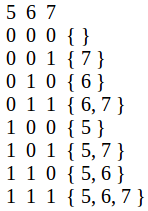Related Articles
Find sum of sum of all sub-sequences
• Difficulty Level : Easy
• Last Updated : 16 Apr, 2020

Given an array of n integers. The task is to find the sum of sum of each of sub-sequence of the array.

Examples :

```Input : arr[] = { 6, 8, 5 }
Output : 76
All subsequence sum are:
{ 6 }, sum = 6
{ 8 }, sum = 8
{ 5 }, sum = 5
{ 6, 8 }, sum = 14
{ 6, 5 }, sum = 11
{ 8, 5 }, sum = 13
{ 6, 8, 5 }, sum = 19
Total sum = 76.

Input  : arr[] = {1, 2}
Output : 6
```

## Recommended: Please try your approach on {IDE} first, before moving on to the solution.

Method 1 (brute force):
Generate all the sub-sequence and find the sum of each sub-sequence.

Method 2 (efficient approach):
For an array of size n, we have 2^n sub-sequences (including empty) in total. Observe, in total 2n sub-sequences, each elements occurs 2n-1 times.
For example, arr[] = { 5, 6, 7 }So, sum of sum of all sub-sequence will be (sum of all the elements) * 2n-1.

Below is the implementation of this approach:

## C++

 `// C++ program to find sum of all sub-sequences ` `// of an array. ` `#include ` `using` `namespace` `std; ` ` `  `// Return sum of sum of all sub-sequence. ` `int` `sum(``int` `arr[], ``int` `n) ` `{ ` `  ``int` `ans = 0; ` ` `  `  ``// Finding sum of the array. ` `  ``for` `(``int` `i = 0; i < n; i++) ` `    ``ans += arr[i]; ` ` `  `  ``return` `ans * ``pow``(2, n - 1); ` `} ` ` `  `// Driver Code ` `int` `main() ` `{ ` `  ``int` `arr[] = { 6, 7, 8 }; ` `  ``int` `n = ``sizeof``(arr)/``sizeof``(arr); ` ` `  `  ``cout << sum(arr, n) << endl; ` ` `  `  ``return` `0; ` `}  `

## Java

 `// Java program to find sum of  ` `// all sub-sequences of an array. ` `import` `java.io.*; ` `import` `java.math.*; ` ` `  `class` `GFG { ` `     `  `    ``// Return sum of sum of all sub-sequence. ` `    ``static` `int` `sum(``int` `arr[], ``int` `n) ` `    ``{ ` `    ``int` `ans = ``0``; ` `     `  `    ``// Finding sum of the array. ` `    ``for` `(``int` `i = ``0``; i < n; i++) ` `        ``ans += arr[i]; ` `     `  `    ``return` `ans * (``int``)(Math.pow(``2``, n - ``1``)); ` `    ``} ` `     `  `    ``// Driver Code ` `    ``public` `static` `void` `main(String args[]) ` `    ``{ ` `    ``int` `arr[]= { ``6``, ``7``, ``8` `}; ` `    ``int` `n = arr.length; ` `     `  `    ``System.out.println(sum(arr, n)); ` `    ``} ` `} ` `     `  `// This code is contributed by Nikita Tiwari. `

## Python3

 `# Python 3 program to find sum of  ` `# all sub-sequences of an array. ` ` `  ` `  `# Return sum of sum of all sub-sequence. ` `def` `sm(arr , n) : ` `    ``ans ``=` `0` ` `  `    ``# Finding sum of the array. ` `    ``for` `i ``in` `range``(``0``, n) : ` `        ``ans ``=` `ans ``+` `arr[i] ` `     `  `    ``return` `ans ``*` `pow``(``2``, n ``-` `1``) ` `     `  `     `  `# Driver Code ` `arr ``=` `[ ``6``, ``7``, ``8` `] ` `n``=``len``(arr) ` ` `  `print``(sm(arr, n)) ` ` `  ` `  `# This code is contributed by Nikita Tiwari. `

## C#

 `// C# program to find sum of  ` `// all sub-sequences of an array. ` `using` `System; ` ` `  `class` `GFG ` `{ ` `     `  `    ``// Return sum of sum of all sub-sequence. ` `    ``static` `int` `sum(``int` `[]arr, ``int` `n) ` `    ``{ ` `    ``int` `ans = 0; ` `     `  `    ``// Finding sum of the array. ` `    ``for` `(``int` `i = 0; i < n; i++) ` `        ``ans += arr[i]; ` `     `  `    ``return` `ans * (``int``)(Math.Pow(2, n - 1)); ` `    ``} ` `     `  `    ``// Driver Code ` `    ``public` `static` `void` `Main() ` `    ``{ ` `    ``int` `[]arr= { 6, 7, 8 }; ` `    ``int` `n = arr.Length; ` `     `  `    ``Console.Write(sum(arr, n)); ` `    ``} ` `} ` `     `  `// This code is contributed by nitin mittal `

## PHP

 ` `

Output:

```84
```

This article is contributed by Anuj Chauhan. If you like GeeksforGeeks and would like to contribute, you can also write an article using contribute.geeksforgeeks.org or mail your article to contribute@geeksforgeeks.org. See your article appearing on the GeeksforGeeks main page and help other Geeks.